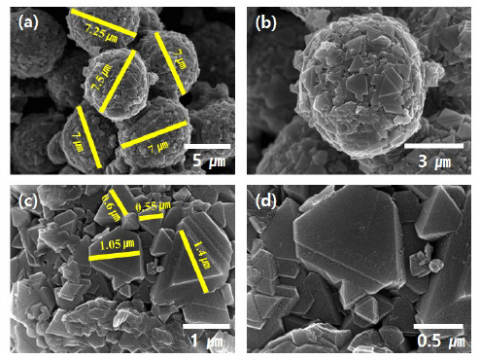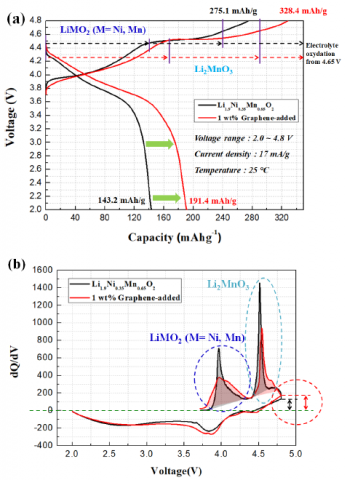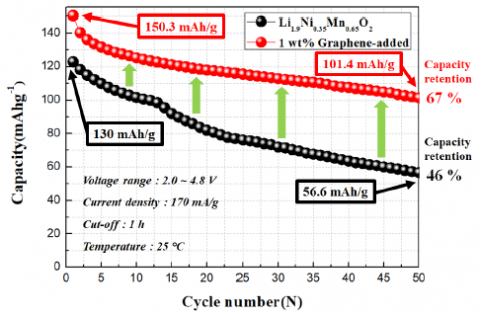# Evaluations of Discharge Capacity and Cycle Stability on Graphene-added Li1.9Ni0.35Mn0.65O2 Cathode by Carbonate Co-precipitation

Evaluations of Discharge Capacity and Cycle Stability on Graphene-added Li1.9Ni0.35Mn0.65O2 Cathode by Carbonate Co-precipitation

Department of Nano-Polymer Science & Engineering, Korea National University of Transportation, Chungju, Chungbuk 380-702, Republic of Korea

Departmeent of Mechanical Engineering, Dong-A University, Busan 49315, Republic of Korea

Department of advanced Materials Engineering, Korea National University of Transportation, Chungju, Chungbuk 380-702, Republic of Korea

Corresponding Author Email:
twhong@ut.ac.kr
Page:
191-194
|
DOI:
https://doi.org/10.14447/jnmes.v22i4.a04
21 December 2018
|
Accepted:
1 April 2019
|
Published:
30 December 2019
| Citation
Abstract:

Li[Li1/3–2x/3Ni xMn2/3–x/3]O2 is currently receiving considerable attention for its use as a modern cathode material for lithium-ion batteries (LIBs), owing to its high capacity of over 250 mAhg-1 when charged to 4.5 V or higher. However, owing to the rapidly fading capacity and poor rate capa- bility of Li[Li1/3–2x/3NixMn2/3–x/3]O2 materials, extensive efforts have been made in recent years to improve their rate capability. In this paper, a Li- rich and manganese-based Li1.9Ni0.35Mn0.65O2 cathode material was prepared by a carbonate co-precipitation method, and graphene, which pro- vides high conductivity and thermal stability, was used during the slurry mixing process. X-ray diffraction (XRD) and scanning electron microsco- py (SEM) were used to characterize their structure and microstructure. The electrochemical properties show that the initial discharge capacity and cycle stability were enhanced due to the high electrical conductivity and thermal stability of graphene.

PACS number: 73.20.At

Keywords:

Mn-rich cathode, Carbonate co-precipitation, Graphene

1. Introduction

High energy and power-density lithium-ion batteries (LIBs) are critically needed for both vehicle and stationary storage applications [1,2]. Unfortunately, the current lithium-ion cathode material does not meet the demands for higher energy and power densities [3,4]. Search-ing for cheaper and safer cathode materials with higher capacity has become a significant subject of research. In this regard, the Li-rich and manganese-based layered solid-solution between layered LiMO2 (M = Ni, Co, and Mn) and layered Li[Li1/3Mn2/3]O2 (commonly designated as Li2MnO3) have become attractive cathode materials owing to their high capacity (>250 mAh g-1), low cost, and enhanced structural stabil-ity [5-7] compared to the commercially available cathodes. Unfortu-nately, these complicated materials are difficult to prepare and they could show low rate capability depending on the synthetic route.

The co-precipitation method [8,9] is an efficient way to produce the current cathode materials, and most of the precursors are synthesized as transition metal hydroxides [10-13]. However, the hydroxide co-precipitation method is not easily controlled for the manganese-rich materials because Mn(OH)2 easily oxidizes to the MnOOH and/or Mn3O4 phases during or after the co-precipitation process, leading to a deviation from the desired stoichiometry, impurities, and low tap den-sity. To solve this problem, a carbonate co-precipitation method [14-16] is suitable to synthesize the transition metal (Ni, Co, Mn) precur-sors. In principle, the carbonate co-precipitation method has the ad-vantage of maintaining the constant oxidation states of Ni, Co, and Mn (equal to 2) in the carbonate matrix . In addition, the experimental conditions of carbonate co-precipitation are less harsh than those of the hydroxide co-precipitation, such as the easy control of the pH value and no need for an inert atmosphere.

Although Li-rich materials have a big advantage over current com-mercial cathode materials with their higher capacity, their low conduc-tivities are considered to be their main drawback, limiting their rate capability and, to some extent, cyclability. There have been numerous attempts to improve Li-rich materials’ rate capability by modifying the grain shape and size, doping, and adding electrically conductive addi-tives [18-22]. As shown further, some experimental works with gra-phene oxide or reduced graphene oxide (rGO) as the conductive addi-tive offer a superior rate capability to those achieved by other methods. However, it is very rare to apply graphene alone to Mn-rich cathode materials prepared by the carbonate co-precipitation method. In this paper, we report the addition of graphene to Li1.9Ni0.35Mn0.65O2 (LMNO), synthesized by carbonate co-precipitation.

2. Experimental

2.1 Synthesis of $\mathbf{L i}_{\mathbf{1} .9} \mathbf{N i}_{\mathbf{0 . 3 5}} \mathbf{C o}_{\mathbf{0 . 6 5}} \mathbf{M} \mathbf{n}_{\mathbf{0 . 1}} \mathbf{O}_{\mathbf{2}}$

[Ni0.35Mn0.65]CO3 powders were prepared by co-precipitation, as previously reported. An aqueous solution of NiSO4 6H2O and MnSO4·H2O was pumped into a continuously stirred tank reactor in an N2 atmosphere. Simultaneously, a Na2CO3 solution and an appropriate amount of NH4OH solution (chelating agent) were fed separately into the reactor. The spherical [Ni0.35Mn0.65]CO3 powders were dried at 130°C for 24 h to remove adsorbed water. Finally, [Ni0.35Mn0.65]CO3, LiOH·H2O was mixed at room temperature for 1 h, then calcined at 950℃ for 24 h in air. The obtained [Ni0.35Mn0.65]CO3 precursor was thoroughly mixed with an appropriate amount of Li2CO3 and calcined at 950℃ for 24 hrs in air.

2.2 Material Characterization

The XRD patterns for the cathodes were obtained using a Siemens D-5000 diffractometer in the 2θ range from 10° to 80° with Cu Kα radiation (λ = 1.54068 Å). The morphology of the obtained powder was observed with SEM (JSM-7610F, JEOL).

2.3 Process of Adding Graphene and Electrochemical Test

To prepare the positive electrode, $80 \% \mathrm{Li}_{1.9} \mathrm{Ni}_{0.35} \mathrm{Mn}_{0.65} \mathrm{O}_{2}$ powder 10 $\%$ super-P carbon black (Aldrich), N-methyl-2-pyrrolidone, 10\% PVDF (Kureha KF100) binder, and 1 wt\% graphene (electrical conductivity $\left.>10^{3} \mathrm{S} / \mathrm{cm}, \text { Aldrich }\right)$ versus the cathode were added to a crucible. After grinding, the viscous slury was coated on aluminum foil using a doctor blade to make a film with a uniform thickness. The film was then dried at $120^{\circ} \mathrm{C}$ for $4 \mathrm{h}$ in a vacuum oven. The CR2032-type coin cell was assembled in a glovebox using the above cathode film, lithi$\mathrm{um},$ a porous polypropylene film, and a $1 \mathrm{M}$ LiPF 6 solution with a 3: 7 volume ratio of ethylene carbonate (EC)/dimethyl carbonate (DMC). The lithium metal foil was used as both the counter and reference electrode. After the coin cell assembly, the test cells were charged and discharged galvano-statically between 2.0 and $4.8 \mathrm{V}$ versus the $\mathrm{Li} / \mathrm{Li}+$, at a constant current density $\left(17 \mathrm{mAg}^{-1} \text {was assumed to be } 0.1 \mathrm{C} \text { rate }\right)$. The cycle performances were carried out at a high current density (170 $\left.\mathrm{mAg}^{-1} \text {was assumed to be } 1 \mathrm{C} \text { rate }\right)$.

3. Results and Discussions

## 1.pngFigure 1. Illustration of the strategy of this study

Figure 1 shows an image of the strategy of this study; $1 .$ Production of Co-free $\mathrm{Li}_{1.9} \mathrm{Ni}_{0.35} \mathrm{Mn}_{0.65} \mathrm{O}_{2}$ cathode materials of cheaper, safer, and higher capacity. 2. Suppression of $\mathrm{MnOOH}$ and $\mathrm{Mn}_{3} \mathrm{O}_{4}$ formation by carbonate co-precipitation, and $3 .$ Enhancement of electrical conductivity and thermal stability of cathode materials using graphene.

## 2.pngFigure 2. XRD patterns of the $\mathrm{Li}_{1.9} \mathrm{Ni}_{0.35} \mathrm{Mn}_{0.65} \mathrm{O}_{2}$

The XRD patterns of LMNO are shown in Fig. 2. As can be seen, $\mathrm{Li}_{1.9} \mathrm{Ni}_{0.35} \mathrm{Mn}_{0.65} \mathrm{O}_{2}$ exhibits layered characteristics and all peaks can be indexed to the hexagonal $\alpha$ -NaFeO $_{2}$ structure (space group: $\mathrm{R} 3 \mathrm{m}$, No. $\mathrm{No}$. 166), except for two peaks (red dashed circles). These peaks may have been caused by $\mathrm{Li}_{2} \mathrm{MnO}_{3}$. Except for $\mathrm{Li}_{2} \mathrm{MnO}_{3}$, the peaks are very sharp, which indicate good crystallinity of the as-prepared materials. In addition, the separations between the adjacent peaks of $(006) /(012)$ and $(018) /(110)$ can be clearly observed, indicating a typical layered structure.

## 3.pngFigure 3. SEM images of the Li1.9Ni0.35Mn0.65O2 particles: (a) X 5000, (b)X 10,000, (c) X 25,000, (d) X 50,000

Figure 3 shows the SEM images of the $\mathrm{Li}_{1.9} \mathrm{Ni}_{0.35} \mathrm{Mn}_{0.65} \mathrm{O}_{2}$ powder. The estimated average particle size was 7 to $8 \mu \mathrm{m},$ and the secondary particles had a spherical morphology, as shown in Figs. 3 (a) and (b). The primary particles of $\mathrm{Li}_{1.9} \mathrm{Ni}_{0.35} \mathrm{Mn}_{0.65} \mathrm{O}_{2}$ were like a triangular prism in shape, with a particle size of 1.4 to $0.55 \mathrm{nm},$ as shown in Figs. 3 (c) and (d), and they were densely agglomerated, producing secondary forms.

The initial charge/discharge curves for the pristine and graphene-added LMNO cells with a constant current density of 17 mA/g (0.1C) cycled in the voltage range of 2.0 and 4.8 V are shown in Fig. 4. As predicted in Fig. 2, a high ratio of the electrochemically inactive Li2M-nO3 plateau was clearly observed in the initial charge curve at all sam-ples, as shown Fig. 4 (a). The dQ/dV plots show that the exact amount of Li2MO3 and LiMO2 can be calculated through integration, as shown Fig. 4 (b). Interestingly, as shown Fig. 4 (b), both samples failed to extract all lithium even at a high voltage of 4.8 V. Despite the high electrical conductivity of the graphene, the graphene-added sample caused less delithiation. This phenomenon is evidence that there was interference with the delithiation. Therefore, it is necessary to confirm this phenomenon through an additional impedance measurement. The initial discharge capacities of the pristine and graphene-added LMNO were 143.2 mAh/g and 191.4 mAh/g, respectively. This enhanced ca-pacity is due to the high electrical conductivity of graphene. In addi-tion, with graphene, the columbic efficient LMNO increased from 52% to 58%. All cells first had an irreversible capacity, of which the voltage plateau was due to the extraction of lithium and oxygen starting at ap-proximately 4.4V, and their columbic efficiency stabilized after the second cycling . The irreversible capacity of all samples probably resulted from the oxygen loss from the structure during first cycling as well as the possible electrolyte decomposition, since the onset potential of the electrolyte is approximately 4.65 V, depending on electrolyte composition . Some portion of the irreversible capacity for all sam-ples in Fig. 4 may come from the electrolyte oxidation. The most irre-versible capacity may be related to the oxygen loss when the cell was charged above 4.45 V . Therefore, the enhanced columbic efficient is due to the high thermal stability of graphene at the high working voltage.

## 4.pngFigure 4. (a) The initial charge/discharge curves and (b) the correspondingdQ/dV plots of the pristine and graphene-added Li1.9Ni0.35Mn0.65O2 at 0.1 C between 2.0 V and 4.8 V at 25°C

## 5.pngFigure 5. Cyclic performances of the pristine and graphene-added Li1.9Ni0.35Mn0.65O2 at 1 C between 2.0 V and 4.8 V at 25°C

Figure 5 shows the cycle performance of the pristine and graphene-added LMNO electrodes at a current density of $170 \mathrm{mA} / \mathrm{g}(1 \mathrm{C})$ from 2.0 V to 4.8 V. The initial discharge capacity of the pristine electrode increased from 123 mAhg $^{-1}$ to 56.6 mAhg $^{-1}$ by the $50^{\text {th }}$ cycle $\left(50^{\text {th }}\right.$ cycle efficiency $=46 \%$ ). The graphene-added LMNO electrode delivered an initial discharge capacity of $150.3 \mathrm{mAhg}^{-1},$ which decreased to 101.4 mAhg $^{-1}$ after the $50^{\text {th }}$ cycle $\left(50^{\text {th }} \text { cycle efficiency }=67 \%\right.$ ). As can be seen in Fig. $5,$ the sample with graphene was found to be more stable than the pristine as the cycle progressed.

## 6.pngFigure 6. Impedance plots of the pristine and graphene-added Li1.9Ni0.35Mn0.65O2

Figure 6 shows the electrochemical impedance spectra for the pris- tine and graphene-added LMNO materials after 1 cycle. Although the graphene had been added, the charge-transfer resistance (Rct) values for these cathode materials increased from 264.8 Ω to 363.4 Ω. This result was predicted due to the increase in electrochemically inactive Li2MnO3, as shown in Fig. 4b (sky color dashed circle). In addition, one plausible reason for this is due to the densely coated graphene-blocking lithium ions, as shown in Fig. 4b (red dashed circle).

4. Conclusions

Li1.9Ni0.35Mn0.65O2 cathode material was prepared by a carbonate co-precipitation method and graphene was used during the slurry mixing process. The XRD results indicated a well indexed α-NaFeO2 structure. However, the peak intensity of Li2MnO3 was slightly larger. The SEM images showed that the Li1.9Ni0.35Mn0.65O2 cathode material size ranged from 7 μm to 8 μm, and the primary particles were triangular-prism-like in shape. The initial discharge capacities of the pristine and gra-phene-added Li1.9Ni0.35Mn0.65O2 were 143.2 mAh/g and 191.4 mAh/g, respectively. The cycle performances of the pristine and graphene-added LMNO electrodes were evaluated at a current density of 170 mA/g (1 C) from 2.0 V to 4.8 V at room temperature. The capacity retention of the pristine and graphene-added Li1.9Ni0.35Mn0.65O2 were 46% to 67% after the 50th cycle, respectively. The graphene-added Li1.9Ni0.35Mn0.65O2 had a significantly improved capacity and cycling stability due to the enhanced electrical conductivity and thermal stability.

Acknowledgment

This study was supported by a grant from the Ministry of Trade In-dustry and Energy of the Republic of Korea (G02N03620000901).

References

 M. Armand, J.-M.Tarascon, Nature, 451, 652 (2008).

 B. Dunn, H. Kamath, J.-M.Tarascon, Science, 334, 928 (2011).

 P.G. Bruce, S.A. Freunberger, L.J. Hardwick, J.M. Tarascon, Nat. Mater., 11, 19 (2011).

 M.M. Thackeray, C. Wolverton, E.D. Isaacs, Energy Environ. Sci., 5, 7854 (2012).

 J.-H. Kim, C.S. Yoon, Y.-K. Sun, J. Electrochem. Soc., 150, 538 (2003).

 C.S. Johnson, J.-S. Kim, C. Lefief, N. Li, J.T. Vaughey, M.M. Thackeray, Electrochem. Commun., 6, 1085 (2004).

 T.A. Arunkumar, E. Alvarez, A. Manthiram, J. Electrochem. Soc., 154, 770 (2007).

 M.E. Spahr, P. Novak, B. Schnyder, O. Haas, R. Nesper, J. Electro-chem. Soc., 145, 1113 (1998).

 C. Storey, I. Kargina, Y. Grincourt, I.J. Davidson, Y.C. Yoo, D.Y. Seung, J. Power Sources, 97-98, 541 (2001).

 M.M. Thackeray, S.-H. Kang, C.S. Johnson, J.T. Vaughey, S.A. Hackney, Electrochem. Commun., 8, 1531 (2006).

 Y. Chen, G.F. Xu, J.L. Li, Y.K. Zhang, Z. Chen, F.Y. Kang, Elec-trochim. Acta, 87, 686 (2013).

 M.-H. Lee, Y.-J.Kang, S.-T.Myung, Y.-K. Sun, Electrochim. Acta, 50, 939 (2004).

 C.S. Johnson, J.-S. Kim, A.J. Kropf, A.J. Kahaian, J.T. Vaughey, L.M.L. Fransson, K. Edstrom, M.M. Thackeray, Chem. Mater., 15, 2313 (2003).

 X.Q. Liu, Z.M. Guo, Prog. Nat. Sci. Mater. Int., 22, 126 (2012).

 J.W. Zhang, X. Guo, S.M. Yao, W.T. Zhu, X.P. Qiu, J. Power Sources, 238, 245 (2013).

 M. Gao, F. Lian, H.Q. Liu, C.J. Tian, L.L. Ma, W.Y. Yang, Elec-trochim. Acta, 95, 87 (2013).

 S.-H. Park, S.-H. Kang, I. Belharouak, Y.K. Sun, K. Amine, J. Power Sources, 177, 177 (2008).

 M. Konarova, I. Taniguchi, J. Power Sources, 195, 3661 (2010).

 Y. Ge, X. Yan, J. Liu, X. Zhang, J. Wang, X. He, R. Wang, H. Xie, Electrochim. Acta, 55, 5886 (2010).

 Y. Yang, X.-Z.Liao, Z.-F.Ma, B.-F. Wang, L. He, Y.-S.He, Elec-trochem. Commun., 11, 1277 (2009).

 X. Li, F. Kang, X. Bai, W. Shen, Electrochem. Commun., 9, 663 (2007).

 B. Huang, X. Zheng, D. Jia, M. Lu, Electrochim. Acta, 55, 1227 (2010).

 D.-K.Lee, S.-H.Park, K.Amine, H.J.Bang, J.Parakash, Y.-K.Sun J. Power Sources, 162, 1346 (2009).

 Z. Lu, J.R. Dahn, J. Electrochem. Soc., 149, 815 (2002).